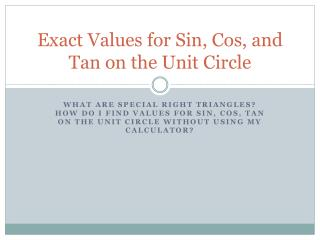Download PresentationExact Values for Sin, Cos, and Tan on the Unit Circle

Loading in 2 Seconds...

# Exact Values for Sin, Cos, and Tan on the Unit Circle - PowerPoint PPT Presentation

Exact Values for Sin, Cos, and Tan on the Unit Circle. What are special right triangles? How do I find values for sin, cos , tan on the unit circle without using my calculator?. Special Right Triangles.I am the owner, or an agent authorized to act on behalf of the owner, of the copyrighted work described.
Download Presentation## Exact Values for Sin, Cos, and Tan on the Unit Circle

An Image/Link below is provided (as is) to download presentation

Download Policy: Content on the Website is provided to you AS IS for your information and personal use and may not be sold / licensed / shared on other websites without getting consent from its author.While downloading, if for some reason you are not able to download a presentation, the publisher may have deleted the file from their server.

- - - - - - - - - - - - - - - - - - - - - - - - - - E N D - - - - - - - - - - - - - - - - - - - - - - - - - -
Presentation Transcript
1. Exact Values for Sin, Cos, and Tan on the Unit Circle What are special right triangles? How do I find values for sin, cos, tan on the unit circle without using my calculator?

2. Special Right Triangles A special right triangleis a right triangle with some regular feature that makes calculations on the triangle easier, or for which simple formulas exist. There are 2 special right triangles and

3. Now lets see how that is useful on the unit circle Remember a unit circle has a radius of 1 Each point on the circle adheres with a specific degree for the circle and at this point there is an exact value for sine and cosine (using radian measure NOT A DECIMAL)

4. Here are the exact values

5. Negative or Positive? All Students Take Chemistry Quadrant II Quadrant I Quadrant IV Quadrant III

6. Larger than 90 degrees If your angle is larger than 90 degrees, find its angle with the x-axis. For example, sin (120 degrees) 60º

7. Example If you don’t have your unit circle memorized yet then convert to degrees Find the reference angle by subtracting 180 Find the exact value on your chart Figure out if negative or positive “All Students Take Chem.”

8. Now get with your group from Thursday and find and label all the exact values for sin and cos